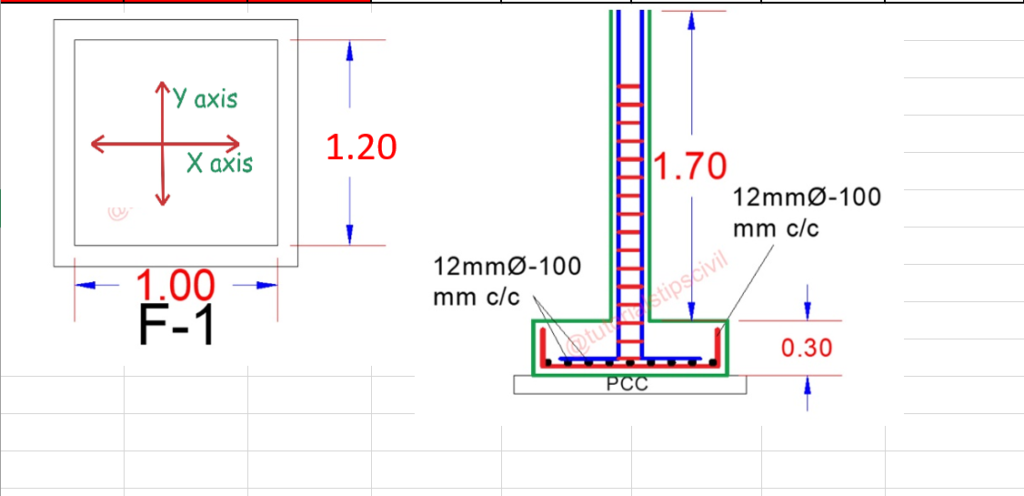# How to calculate BBS of Rectangular Footing with few Easy Step

### How to make BBS on Rectangular Footing.

Calculate BBS of Footing Just 4 Step

Given Data From Footing Drawing

Footing Size  = 1 m x 1.2 m
Spacing of Bar  = 100 mm C/c
Footing Cover  = 50 mm
Bar Use in Footing = 12 mmStep- 1
Cutting Length of Y-Axis

Cutting Length = Length (Y-Axis) – (2x Cover) + (2x Height)  – 4 Cover (For  “L” Upside)  – Bend

Cutting Length = {1200 – (2 x 50) }+ (2 x 300) – (4 x 50) – (2 x 2 x 12)

Cutting Length = 1100 + 600 – 200 – 48

Cutting Length = 1100 + 600 – 248

Cutting Length = 1700 – 248

Cutting Length = 1452

Cutting Length = 1.45 m

Step- 2
Cutting Length of X-Axis

Cutting Length = Length (X-Axis) – (2x Cover) + (2x Height)  – 4 Cover (For  “L” Upside)  – Bend

Cutting Length = {1000-(2 x 50)} + (2 x 300) – (4 x 50) – (2 x 2 x 12)

Cutting Length = 900 + 600 – 200 – 48

Cutting Length = 900 + 600 – 248

Cutting Length = 1500 – 248

Cutting Length= 1252

Cutting Length= 1.25 m

Step- 3
Numbers of bar Along With X Axis ( Length – 1.45 m)

Number of Bar X-Axis = [ Length – (2x Cover) ] /Spacing + 1

Number of Bar X-Axis = [ 1000 – (2x 50) ] /100 + 1

Number of Bar X-Axis = ( 900 / 100 ) + 1

Number of Bar X-Axis = 10 Nos

We Need 10 Number of bar for length of 1.45 m.

Step- 4
Numbers of bar Along With Y Axis ( Length – 1.25 m)

Number of Bar X-Axis = [ Length – (2x Cover) ] /Spacing + 1

Number of Bar X-Axis = [ 1200 – (2x 50) ] /100 + 1

Number of Bar X-Axis = ( 1100 / 100 ) + 1

Number of Bar X-Axis = 12 Nos

We Need 12 Number of bar for length of 1.25 m.